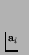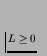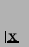Next: Inter-Organism Communications Up: The Environment Previous: Private Energy.

## Moving around the Grid

A cell does not have to remain in its original grid position, but can move around by using the move instruction. The contents of the cx register at the time the instruction is issued determines in which direction the cell will try to move (the low 3 bits specify a direction from 0 to 7, as indicated in Figure 4.3(a)).

However, movement is complicated by the fact that a cell may be part of a multicellular organism (in which other cells are also trying to move, possibly in different directions). The organism must move as a whole, so what actually happens is that the issuing of a move instruction by a cell is actually a vote to move in a particular direction rather than an instruction that has immediate effect. At each time slice, an organism counts up all of the movement votes from its constituent cells, and decides how to move as follows:

A normalised total movement vectoris calculated by summing all the individual votes of cells within the organism:

 (4.1)

where n is the total number of cells in the organism, andis the unit vector of movement (in one of eight possible directions) specified by cell i (or if the cell did not issue a move instruction during the current time slice).

A multiple movement factor', M, is then calculated. This factor determines the extent to which two or more cells moving in tandem within an organism are more efficient than would be expected by simply summing their individual movements. M is defined as:

 M = (m-1)L+1 (4.2)

where m is the number of cells within the organism that individually issued a move instruction; and L is the constant of leverage' when two or more cells move at the same time (). L is defined by the global parameter movement_leverage_factor.

Movement is further complicated in the situation where the organism is overlapping (or partially overlapping) another organism on the grid. In this case, there is a `friction' term F which slows the organism down as it attempts to move over other cells. This term is defined as follows:

 (4.3)

where o is the number of cells in the organism which share a grid position with cell(s) from other organisms. The friction factor can actually be turned on or off with the global parameter apply_friction_factor. If it is turned off, F is effectively set to zero.

The total movement that the organism attempts to make,, is therefore specified by

 (4.4)

The organism moves from its current position by the distance and direction given by, unless it reaches the edge of the grid, in which case it stops at that point (if the grid boundary does not wrap).Next: Inter-Organism Communications Up: The Environment Previous: Private Energy.
Tim Taylor
1999-05-29